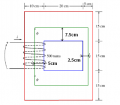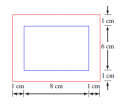# Mean Path Length of a Magnetic core????

#### TheSpArK505

Joined Sep 25, 2013
126
Hello everyone.

I,m studying magnetic circuits and I'm stuck in calculating the mean path length of a squared core and circular one.I can't upload the circuits due to some issues in my PC.
If any one could explain any circuit and generalize the idea that would be great.

waiting for responds......thanks.

#### TheSpArK505

Joined Sep 25, 2013
126
if u have a specific article or explanation on effective mean path length calculations or derivations that would be helpful....Waiting for that.#### Kermit2

Joined Feb 5, 2010
4,162The conversion factor derivation for the ancient units of magnetisn are briefly explained in the last paragraph. The values
you calculate can be converted online with the help of google etc. So, what more do you
need to help you. posting an example of the problem you are working on and where
and costs you REAL money.

#### Jony130

Joined Feb 17, 2009
5,351
Effective mean path length for this core is ??The red line represents the longest path which a flux line could take to go round the core. The blue line is the shortest path. And in green is the mean length.
le = (l_red + l_blue)/2
l_red = 2*(10cm + 20cm + 5cm) +2*(15cm + 15cm + 15cm) = 160cm
l_blue = 2*20cm + 2*15cm = 70cm
le = (160cm + 70cm)/2 = 115cm

And for this corelef = 32cm

#### Syed Kawish Quyyum

Joined Sep 12, 2015
1
Effective mean path length for this core is ??

View attachment 74327

The red line represents the longest path which a flux line could take to go round the core. The blue line is the shortest path. And in green is the mean length.
le = (l_red + l_blue)/2
l_red = 2*(10cm + 20cm + 5cm) +2*(15cm + 15cm + 15cm) = 160cm
l_blue = 2*20cm + 2*15cm = 70cm
le = (160cm + 70cm)/2 = 115cm

And for this core
View attachment 74328

lef = 32cm
Thanks Dude. It really helped